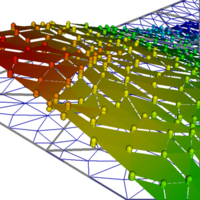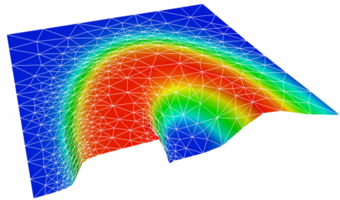You're not currently signed in.# Numerical Methods for Partial Differential Equations

## CS555 :: Spring 2018

This course covers the basics of finite difference schemes, finite volume schemes, and finite element methods (majority). In addition, we'll cover some advanced topics such as discontinuous Galerkin and spectral methods.

One of the goals of this course is to build intuition for these methods. We'll be using Python and will be providing background for many of the computational and mathematical concepts in the course. As such, you do not need to be an expert in PDEs or in coding. But you should have a course in numerical analysis as your background (CS450 or equivalent), be comfortable with differential equations, and have some coding experience.

The course is divided in roughly two parts: advection and elliptic. This is of course a generalization, but it does allow us to focus on finite difference/finite volume methods for one part of the course and finite elements for another part. In addition to model problems we'll look at Stokes and other equations in order to develop a full understanding of the methods.

The course involves several homeworks (usually bi-weekly) and two projects: a midsemester project and a final project. There is also a participation grade based on quizzes.

The course homeworks and examples in class will be in Python. In particular, we'll use numpy and scipy. (Some early examples will be in Matlab.)

- Homeworks (40)